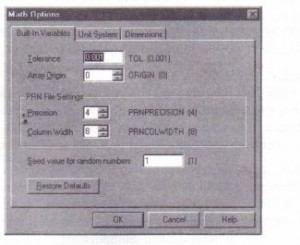Mathcad includes several variables that, unlike ordinary variables, are already defined when you start Mathcad. These variables are called predefined or built-in variables. Predefined variables either have a conventional value, like 1t and e, or are used as system
variables to control how Mathcad works, like ORIGIN and TaL.Definition and use Pi. Mathcad uses the value of1t to 15 digits in numerical calculations. In symbolic calculations, 1t is exact. To
type 1t, press [Ctrl]P. The base of natural logarithms. Mathcad uses the value of e to 15 digits in numerical calculations. In symbolic calculations, e is exact. Infinity. In numerical calculations, this is an actual number of finite magnitude. In symbolic calculations, this represents a true infinity. To type », press [Ctrl]Z. Percent. Use this in expressions like 10 . % or as a scaling unit in the placeholder at the end of an equation with an equals sign. Numerical tolerance for the various approximation algorithms (integrals, equation solving, etc.). See the sections on the specific operations for details. Array origin: the index of the first element of an array. Column width used when writing files with the WRITEPRN function. Number of significant digits used when writing files with the WRITEPRN function. Used to drive animation. Set to zero when no animation is in progress. String corresponding to the directory of the worksheet.
Input and output variables (inO, inl, outO, outl, etc.) in a Mathcad component in a MathConnex system. See the MathConnex Getting Started Guide for details In addition to the predefined variables in the table above, Mathcad treats the names of all built-in units as predefined variables. See Chapter 9, “Units and Dimensions,”for more on using units in your calculations; a list of built-in units is in Appendix B, “Unit Tables.” Although Mathcad’ s predefined variables already have values when you start Mathcad, you can still redefine them. For example, if you want to use a variable called e with a value other than the one Mathcad provides, enter a new definition, like e := 2 . The variable e takes on the new value everywhere in the worksheet below the new definition. Mathcad’s predefined variables are defined for all fonts, sizes, and styles. This means   that if you redefine e as shown above, you can still use e, for example, as the base for natural logarithms. Note, however, that Greek letters are not included. This means that “E”, although it is typed as “e” in the Symbol font, is not the same number as e. You can control the values of TOL, ORIGIN, PRNPRECISION, and PRNCOL WIDTH without having to explicitly define them in your worksheet. To do so, choose Options from the Math menu, and click on the Built-In Variables tab, as shown belowTo set new starting values for any of these variables, enter a new value in the appropriate text box and click “OK.” Then choose Calculate Worksheet from the Math menu to ensure that all existing equations take the new values into account The numbers in brackets to the right of the variable names represent the default values for those variables. To restore these default values, click on the “Restore Defaults” button and then click “OK.” Chemistry notation Ordinarily, a name cannot contain other operators within it. There may be times, however, when you want to define a name which contains superscripts, subscripts, or other operators as part of it. For example, you may want assign a value to the variable named H20. To do this:
• Press [Ctrl][Shift]O. This inserts a pair of brackets with a placeholder be- I [.!Il I tween them.
‘. • Type H[2.

• Press the [Space] key to place the H2 between the editing lines.
• Now type O

Posted on November 21, 2015 in Variables and Constants